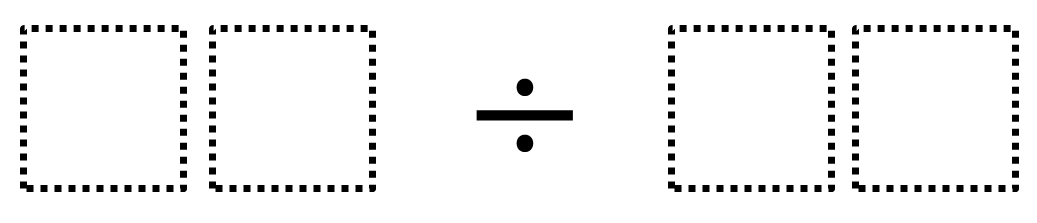Home > Grade 4 > Dividing Two-Digit Numbers (Elementary)

# Dividing Two-Digit Numbers (Elementary)

Directions:  Make the smallest (or largest) quotient by filling in the boxes using the whole numbers 1-9 no more than one time each### Hint

What does the number on the left represent?  What does the number on the right represent?

Answers will vary depending on whether decimal answers are allowed. 98 ÷ 12 is one answer for the largest quotient.  12 ÷ 98 is the smallest quotient.

Source:  Robert Kaplinsky

## Multiplying Differences

Directions: Using the digits 1 to 9, at most one time each, fill in the …

1.I changed it a little bit. Make the largest quotient by filling in the boxes using whole numbers 1 – 9 no more than one time each. No remainders. I know using 98 as the dividend will be what I want to use. I can’t use 12 or 13 as the divisor. Wait a minute I can use 14 as the divisor. That’s the answer 98 divided by 14 equals 7.

Any thoughts.

•I like this version, too. You could also make the smallest quotient possible, not including quotients less than one. The smallest whole number quotient possible will be 2, given that you can’t repeat any of the digits, and there are actually many ways (I counted 21) of getting 2 as the quotient of two 2-digit numbers using the digits 1-9 no more than one time each. Students could try to find them all, or you could limit the digits to 1 through 6, and then there are a lot fewer solutions (I counted 4).

2.my students discovered that 96 divided by 12 is 8 therefore making it larger than your answer of 7. GO WAMPUS CATS!

3.Yeah, lots of answers are possible depending on what assumptions you make. For example, Miss Jackson’s 96 divided by 12 appears to be the largest whole number quotient. If you allow for decimals, that does change things. Well done everyone!

4.98 / 11 = 8.90909091 is bigger than 8. P.S. Chinstrap Penguins are the best.

•However, you used two of the same digit but I would definitely commend a student for making that attempt.